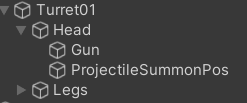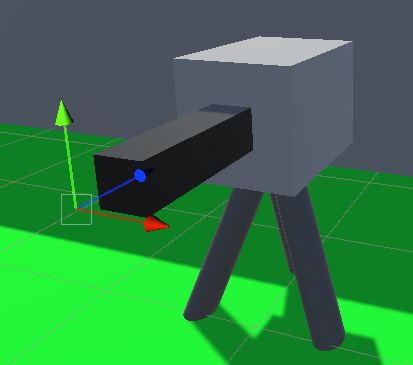# Calculating movement for a projectile based on angle

I am a biginner to unity but I have experience in python programming (and a little bit of java programming as well) so I tried to do this on my own. My code always misses when you aren’t near to the turret. I tripple checked if any input variables weren’t garbage and all variables and numbers are correct. The calculations are for 2D space because my 3D game objects don’t change the y-axis so x-z axis are enought for the turret to hit the player. The `ProjectileHandler` class is responsible for the movementand I think it is working correctly. (the movement is just constant movement in the dirrection provided, it doesn’t slow down or change the `speedX` and `speedY` variables) The variable `projectileMovement` is refering to how fast the projectile should move on the x-z axis in order to hit the player if the player is standing still. The angle for movement calculations is from the turret part that points towards the player. It is actually pointing in the correct direction and it doesn’t have the offset that the fired projectile has. I have been working on this for 2 days now and can’t fix this. Any help would be appreciated. Also this is my first question and I don’t know if I did everithing correctly or if I typed to much so if I did something wrong please tell me.

Code for summoning projectiles:

``````using System.Collections;
using System.Collections.Generic;
using UnityEngine;

public class TurretGunHandler : MonoBehaviour
{
public GameObject gun;
public GameObject player;
public GameObject projectile;
public GameObject ProjectileSummonPosObject;
public float projectileDamage;
public float projectileSpeed;
private static Transform playerT;
private Transform turretT;
private Transform legsT;
private Transform gunT;
private Transform summonT;
private float lastFireT;

void Start()
{
playerT = player.GetComponent<Transform>();
turretT = gameObject.GetComponent<Transform>();
gunT = gun.GetComponent<Transform>();
summonT = ProjectileSummonPosObject.GetComponent<Transform>();
lastFireT = Time.time+Random.Range(0, 1);
}

void SummonProjectile(Vector2 projectileMovement) {
GameObject clone = Instantiate(projectile, summonT.position, playerT.rotation);
ProjectileHandler p = clone.GetComponent<ProjectileHandler>();
p.speedX = projectileMovement.x;
p.speedY = projectileMovement.y;
p.dmg = projectileDamage;
}

void Update()
{
// Rotate turret towards the player
Quaternion prevRotation = turretT.rotation;
turretT.LookAt(playerT);
turretT.Rotate(new Vector3(0, 180, 0));
turretT.rotation = new Quaternion(prevRotation.x, turretT.rotation.y, prevRotation.z, turretT.rotation.w);
// Fire projectile
float angle;
if(turretT.eulerAngles.y <= 180f) {
angle = turretT.eulerAngles.y / 180 * -2;
}
else {
angle = (turretT.eulerAngles.y - 360f) / 180 * -2;
}

Vector2 movement = new Vector2(projectileSpeed*angle, Mathf.Abs(angle));
if (movement.y != angle) {
movement.y = projectileSpeed*(1+angle)*-1;
} else {
movement.y = projectileSpeed*(1-angle)*-1;
}

Debug.Log(movement.ToString());
Debug.Log(angle);

SummonProjectile(movement);
lastFireT = Time.time;
}
}
}
``````

Movement part of `ProjectileHandler` class (Also the only usage of `speedX` and `speedY` variables):

``````void Update()
{
rigid.velocity = new Vector3(speedX, 0, speedY);
}
``````

This is the turret structure and how it looks (The highlighted object is `ProjectileSummonPos` and it is in line with the firing barrel (Firing barrel is called `Gun`) with is always pointing exactly towards the player):